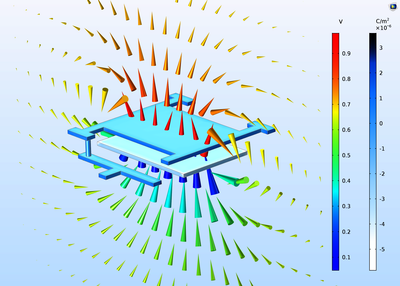# アプリケーションギャラリ

## Tunable MEMS Capacitor

Application ID: 123

The electrostatically tunable parallel plate capacitor in this example is a typical component in various MEMS devices for radio frequencies that range between 300 MHz and 300 GHz. You can modify the distance between the two plates, as the applied voltage changes, through a spring attached to one of the plates. A postprocessing step then computes the capacitance.

The example uses the Electrostatics, Boundary Elements interface, which is based on the boundary element method (BEM), for this simulation. This interface negates the need for defining a finite modeling domain and boundary as well as meshing the thin volume of the capacitor.This model example illustrates applications of this type that would nominally be built using the following products: